NiveditaGachibowli P Janardhan Reddy Nagar, Shamshabad, India - 500081# Nivedita## Experience and Certified Math and Science Tutor With...

Gachibowli P Janardhan Reddy Nagar, Shamshabad, India - 500081.

5.0

UrbanPro Rating

Details verified of Nivedita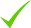IdentityEducation

Know how UrbanPro verifies Tutor details

Identity is verified based on matching the details uploaded by the Tutor with government databases.

## Overview

I am a engineer. I am giving home and online tuition since 2014. I am certified in mathematics, science. I have a degree in electronics and communication engineering. My skills are Excel,vlsi,C programming. I also taught Sanskrit because it was one of my subject from class 9-12.

## Languages Spoken

Hindi Mother Tongue (Native)

English Proficient

## Education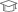Vinobha bhave University 2017

Bachelor of Technology (B.Tech.)

Gachibowli P Janardhan Reddy Nagar, Shamshabad, India - 500081

## Verified Info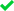ID VerifiedEducation VerifiedPhone VerifiedEmail Verified

## Demo Class

Yes, not charged

Report this Profile

Is this listing inaccurate or duplicate? Any other problem?

Please tell us about the problem and we will fix it.

Please describe the problem that you see in this page.

Type the letters as shown below *

Please enter the letters as show below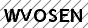## Class 10 Tuition Overview

Class 10 Tuition

Class Location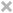Student's HomeTutor's HomeOnline (video chat via skype, google hangout etc)

Years of Experience in Class 10 Tuition

3

Board

CBSE

CBSE Subjects taught

Science, Mathematics, Sanskrit

Experience in School or College

I have completed b.tech in electronics and communication engineering.My teaching experience at Urban elementary school has given me an appreciation for the challenges which children in setting face.I learned how to identify and respond to the various learning styles of different children.Developed lesson plan for 9,10,11,12 maths,sci.students.Established reward system to manage behavior, resulting in marked decrease in detentions.I also taught in college during my study.

Taught in School or College

Yes

## Reviews

this is test message this is test message this is test message this is test message this is test message this is test message this is test message

No Reviews yet! Be the first one to Review

## FAQs

1. Which classes do you teach?

I teach BTech Tuition, Class 10 Tuition, Class 11 Tuition, Class 12 Tuition and Class I-V Tuition Classes.

2. Do you provide a demo class?

Yes, I provide a paid demo class.

3. How many years of experience do you have?

I have been teaching for 3 years.

## Answers by Nivedita (7)

Answered on 17 Aug CBSE/Class 9/Mathematics/Unit 6-Statistics and Probability/Probability/NCERT Solutions/Exercise 15.1

Eleven bags of wheat flour, each marked 5 kg, actually contained the following weightsof flour (in kg):... ...more
Eleven bags of wheat flour, each marked 5 kg, actually contained the following weights
of flour (in kg): 4.97 5.05 5.08 5.03 5.00 5.06 5.08 4.98 5.04 5.07 5.00
Find the probability that any of these bags chosen at random contains more than 5 kg
of flour.

Total number of bags=11 Number of bags containing more than 5kg of flour=7(5.05,5.08,5.03,,5.06,5.08,5.04,5.07) Now, Probability of bags chosen= Number of bags with more than 5kg/Total number of bags =7/11
Dislike Bookmark

Answered on 17 Aug CBSE/Class 9/Mathematics/Unit 6-Statistics and Probability/Statistics/NCERT Solutions/Exercise 14.4

Mean=(2+3+4+5+0+1+3+3+4+3)/10 =2.8 For median, Rewrite list in numerical order, 0,1,2,3,3,3,3,4,4,5 There are 10 numbers,so middle one=(10+2)/2 =6 So,median is 3 Mode is also 3( because this number is repeated more often than other) ...more

Mean=(2+3+4+5+0+1+3+3+4+3)/10

=2.8

For median,

Rewrite list in numerical order,

0,1,2,3,3,3,3,4,4,5

There are 10 numbers,so middle one=(10+2)/2

=6

So,median is 3

Mode is also 3( because this number is repeated more often than other)

Dislike Bookmark

Answered on 17 Aug CBSE/Class 9/Mathematics/Unit 6-Statistics and Probability/Statistics/NCERT Solutions/Exercise 14.4

Mean=(41+39+48+52+46+62+54+40+96+52+98+40+42+52+60)/15 =822/15 =54.8 For median, Rewrite list in numerical order 39,40,40,41,42,46,48,52,52,52,54,60,62,96,98 There are 15 numbers,so middle one,(15+1)/2=8 , So,median is 52 Mode is also 52( because this no.is repeated more often than other) ...more

Mean=(41+39+48+52+46+62+54+40+96+52+98+40+42+52+60)/15

=822/15

=54.8

For median,

Rewrite list in numerical order

39,40,40,41,42,46,48,52,52,52,54,60,62,96,98

There are 15 numbers,so middle one,(15+1)/2=8 ,

So,median is 52

Mode is also 52( because this no.is repeated more often than other)

Dislike Bookmark

Answered on 17 Aug CBSE/Class 9/Mathematics/Unit 6-Statistics and Probability/Statistics/NCERT Solutions/Exercise 14.4

Find the mode of 14, 25, 14, 28, 18, 17, 18, 14, 23, 22, 14, 18.

Here mode is 14 Because mode is the number that is repeated more often than any other.
Dislike Bookmark

Answered on 17 Aug CBSE/Class 9/Mathematics/Surface Area and Volumes/NCERT Solutions/Exercise 13.7

(1)- Given, radius of a cone, r =7cm,and Slant height, l =25cm Let the height of the cone be =h cm Since,we know that Height (h) of cone Now, Capacity of a vessel=volume of cone Volume of cone Therefore, capacity of the conical vessel = = 1.232 litres (2)Given, Height,h= 12cm,and Slant height,... ...more

(1)- Given,

radius of a cone, r =7cm,and

Slant height, l =25cm

Let the height of the cone be =h cm

Since,we know that

Height (h) of coneNow,

Capacity of a vessel=volume of cone

Volume of coneTherefore, capacity of the conical vessel= 1.232 litres

(2)Given,

Height,h= 12cm,and

Slant height, l =13cm

Let,radius of cone = r cm

Since we know that

Radius (r) of coneVolume of coneTherefore, capacity of the conical vessellitres

Dislike Bookmark

x
Class 10 Tuition 5.0

Class LocationStudent's HomeTutor's HomeOnline (video chat via skype, google hangout etc)

Years of Experience in Class 10 Tuition

3

Board

CBSE

CBSE Subjects taught

Science, Mathematics, Sanskrit

Experience in School or College

I have completed b.tech in electronics and communication engineering.My teaching experience at Urban elementary school has given me an appreciation for the challenges which children in setting face.I learned how to identify and respond to the various learning styles of different children.Developed lesson plan for 9,10,11,12 maths,sci.students.Established reward system to manage behavior, resulting in marked decrease in detentions.I also taught in college during my study.

Taught in School or College

Yes

Class 11 Tuition 5.0

Class LocationStudent's HomeTutor's HomeOnline (video chat via skype, google hangout etc)

Years of Experience in Class 11 Tuition

3

Board

CBSE

CBSE Subjects taught

Physics, Chemistry, Mathematics

Taught in School or College

Yes

Class 12 Tuition 5.0

Class LocationStudent's HomeTutor's HomeOnline (video chat via skype, google hangout etc)

Years of Experience in Class 12 Tuition

3

Board

CBSE

CBSE Subjects taught

Physics, Chemistry, Mathematics

Experience in School or College

Explained mathematics and physics concepts in easy to understand language,earned numerous recommendation from students and parents .

Taught in School or College

Yes

BTech Tuition 5.0

Class LocationStudent's HomeTutor's HomeOnline (video chat via skype, google hangout etc)

Years of Experience in BTech Tuition

3

BTech Electrical & Communication

Wireless Communication, Digital Electronics

BTech Branch

BTech 1st Year Engineering, Telecom Engineering, BTech Electrical & Communication Engineering

Experience in School or College

Succedded in helping sudents raise their grade an average of 45%,interacted with other teachers to develop curriculum and practice tests for student, explained mathematics and physics concepts in easy to understand language,analyse students comprehension and prepared goals,earned numerous recommendation from students and parents.

Type of class

Regular Classes

Class strength catered to

One on one/ Private Tutions

Taught in School or College

Yes

BTech 1st Year subjects

Engineering Mathematics (M1), Basic Electronics

Class I-V Tuition 5.0

Class LocationStudent's HomeTutor's HomeOnline (video chat via skype, google hangout etc)

Years of Experience in Class I-V Tuition

4

Board

ICSE, CBSE

CBSE Subjects taught

Science, EVS, Hindi, English, Computers, Mathematics, Sanskrit, Social Science

ICSE Subjects taught

Social Studies, Sanskrit, EVS, English, Mathematics, Hindi, Computer science, Science

Experience in School or College

Integrated reading and writing to offer new perspective and respect for student's own life stories. Monitored, assessed, and remediated student performance Developed and implemented comprehensive lesson plans that accommodated specific level of learners while offering support to make certain each student was able to grasp materials

Taught in School or College

Yes

Teaching Experience in detail in Class I-V Tuition

taught with unique, innovative and interesting methodologies so that students can learn without feeling any burden or boredom.

this is test message this is test message this is test message this is test message this is test message this is test message this is test message

No Reviews yet! Be the first one to Review

## Answers by Nivedita (7)

Answered on 17 Aug CBSE/Class 9/Mathematics/Unit 6-Statistics and Probability/Probability/NCERT Solutions/Exercise 15.1

Eleven bags of wheat flour, each marked 5 kg, actually contained the following weightsof flour (in kg):... ...more
Eleven bags of wheat flour, each marked 5 kg, actually contained the following weights
of flour (in kg): 4.97 5.05 5.08 5.03 5.00 5.06 5.08 4.98 5.04 5.07 5.00
Find the probability that any of these bags chosen at random contains more than 5 kg
of flour.

Total number of bags=11 Number of bags containing more than 5kg of flour=7(5.05,5.08,5.03,,5.06,5.08,5.04,5.07) Now, Probability of bags chosen= Number of bags with more than 5kg/Total number of bags =7/11
Dislike Bookmark

Answered on 17 Aug CBSE/Class 9/Mathematics/Unit 6-Statistics and Probability/Statistics/NCERT Solutions/Exercise 14.4

Mean=(2+3+4+5+0+1+3+3+4+3)/10 =2.8 For median, Rewrite list in numerical order, 0,1,2,3,3,3,3,4,4,5 There are 10 numbers,so middle one=(10+2)/2 =6 So,median is 3 Mode is also 3( because this number is repeated more often than other) ...more

Mean=(2+3+4+5+0+1+3+3+4+3)/10

=2.8

For median,

Rewrite list in numerical order,

0,1,2,3,3,3,3,4,4,5

There are 10 numbers,so middle one=(10+2)/2

=6

So,median is 3

Mode is also 3( because this number is repeated more often than other)

Dislike Bookmark

Answered on 17 Aug CBSE/Class 9/Mathematics/Unit 6-Statistics and Probability/Statistics/NCERT Solutions/Exercise 14.4

Mean=(41+39+48+52+46+62+54+40+96+52+98+40+42+52+60)/15 =822/15 =54.8 For median, Rewrite list in numerical order 39,40,40,41,42,46,48,52,52,52,54,60,62,96,98 There are 15 numbers,so middle one,(15+1)/2=8 , So,median is 52 Mode is also 52( because this no.is repeated more often than other) ...more

Mean=(41+39+48+52+46+62+54+40+96+52+98+40+42+52+60)/15

=822/15

=54.8

For median,

Rewrite list in numerical order

39,40,40,41,42,46,48,52,52,52,54,60,62,96,98

There are 15 numbers,so middle one,(15+1)/2=8 ,

So,median is 52

Mode is also 52( because this no.is repeated more often than other)

Dislike Bookmark

Answered on 17 Aug CBSE/Class 9/Mathematics/Unit 6-Statistics and Probability/Statistics/NCERT Solutions/Exercise 14.4

Find the mode of 14, 25, 14, 28, 18, 17, 18, 14, 23, 22, 14, 18.

Here mode is 14 Because mode is the number that is repeated more often than any other.
Dislike Bookmark

Answered on 17 Aug CBSE/Class 9/Mathematics/Surface Area and Volumes/NCERT Solutions/Exercise 13.7

(1)- Given, radius of a cone, r =7cm,and Slant height, l =25cm Let the height of the cone be =h cm Since,we know that Height (h) of cone Now, Capacity of a vessel=volume of cone Volume of cone Therefore, capacity of the conical vessel = = 1.232 litres (2)Given, Height,h= 12cm,and Slant height,... ...more

(1)- Given,

radius of a cone, r =7cm,and

Slant height, l =25cm

Let the height of the cone be =h cm

Since,we know that

Height (h) of coneNow,

Capacity of a vessel=volume of cone

Volume of coneTherefore, capacity of the conical vessel= 1.232 litres

(2)Given,

Height,h= 12cm,and

Slant height, l =13cm

Let,radius of cone = r cm

Since we know that

Radius (r) of coneVolume of coneTherefore, capacity of the conical vessellitres

Dislike Bookmark

Nivedita describes herself as Experience and Certified Math and Science Tutor With 3 Year of Experience. She conducts classes in BTech Tuition, Class 10 Tuition and Class 11 Tuition. Nivedita is located in Gachibowli P Janardhan Reddy Nagar, Shamshabad. Nivedita takes Regular Classes- at her Home and Online Classes- via online medium. She has 4 years of teaching experience . Nivedita has completed Bachelor of Technology (B.Tech.) from Vinobha bhave University in 2017. She is well versed in English and Hindi.

Recommended Profiles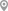Banashankari 1st Stage, BangaloreSaroornagar Sri Venkateshwara Colony, ShamshabadGachibowli P Janardhan Reddy Nagar, ShamshabadGachibowli P Janardhan Reddy Nagar, ShamshabadNacharam Industrial Development Area, ShamshabadWhitefield, Bangalore

X

Post your Learning Need

Let us shortlist and give the best tutors and institutes.

or

Send Enquiry to Nivedita

Let Nivedita know you are interested in their class

Reply to 's review